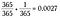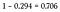# The Birthday Problem🎈

Today’s problem goes out to a special new member of the family. Welcome to the world my niece, Edison Grace Berry! My brother’s beautiful baby girl was born on his 36th birthday this past Saturday and of course this coincidence made me think of the Birthday Problem.

So here it is: a special math problem for a special little girl. Someday you’ll know all the math to understand this post (trust me, I’ll make sure of it!).

→ For more math tutorials, check out Math Hacks on YouTube!

# The Birthday Problem in Real Life

The first time I heard this problem, I was sitting in a 300 level Mathematical Statistics course in a small university in the pacific northwest. It was a class of about 30 students and the professor bet that at least two of us shared the same birthday.

He then proceeded to have everyone state their birthday. When it came to my turn I stated my birthdate as “two cubed, three cubed,” which made the class laugh as our cerebral professor took awhile to decipher the date.

Anyway like he predicted before he got to the last student a pair of matching birthdays had been found.

So how lucky was it that he found a matching pair?

# Warm-Up

Assumption: for the sake of simplicity we’ll ignore the possibility of being born on Feb. 29th.

Let’s begin with a simple example to warm up our brains:

What is the probability that two people share the same birthday?

Person A can be born on any day of the year since they’re the first person we’re asking. The probability of being born any day of the year is 1 or more specifically: 365/365.

Since Person B must be born on the same day as Person A their probability is 1/365.

We want both of these events to happen so multiply the probabilities:The probability that any randomly chosen 2 people share the same birthdate.

So you have a 0.27% chance of walking up to a stranger and discovering that their birthday is the same day as yours. That’s pretty slim.

But what about a larger group?

What’s the chance that at least 2 out of 4 people share the same birthday?

Well to solve this problem we’d have to calculate all of the following:

• Probability A and B share the same birthday
• Probability A and C share the same birthday
• Probability A and D share the same birthday
• Probability B and C share the same birthday
• Probability B and D share the same birthday
• Probability C and D share the same birthday
• Probability A, B and C share the same birthday
• Probability B, C and D share the same birthday
• Probability A, C and D share the same birthday
• Probability A, B and D share the same birthday
• Probability A, B, C and D all share the same birthday

Yuck, that’s a lot of calculations! Imagine how many probabilities we’d have to calculate for a classroom of 30 students!

There’s gotta be a better way…

# A Better Way: the Trick of the Complement

The simplest way of getting around calculating a bajillion probabilities is to look at the problem from a different angle:

What’s the probability that no one shares the same birthday?

This alternate exercise is helpful because it is the complete opposite of our original problem (i.e. the complement). In probability, we know that the total of all the possible outcomes (i.e. the sample space) is always equal to 1, or 100% chance.

Since the probability of at least 2 people having the same birthday and the probability of no one having the same birthday cover all possible scenarios, we know that the sum of their probabilities is 1.

Or equivalently:

Yay! That’ll be much easier to calculate.

# The Calculation

Awesome! We’re finally ready to find out how safe a bet the professor made.

Let’s work out the probability that no one shares the same birthday out of a room of 30 people.

Let’s take this step by step:

• The first student can be born on any day, so we’ll give him a probability of 365/365.
• The next student is now limited to 364 possible days, so the second student’s probability is 364/365.
• The third student may be born on any of the remaining 363 days, so 363/365.

This pattern continues so that our last student has a probability of 336/365 (365 – 29 days since the students before her used up 29 potential days).

Again multiply all 30 probabilities together:

Hold up! That’s a little messy. Let’s clean this up.

Since the denominator is thirty 365’s multiplied together, we could rewrite it as:

Let’s use factorials (symbolically: !) to further clean this calculation up.

(Remember factorials are handy for multiplying together descending positive integers. For example 5! equals 5•4•3•2•1 = 120.)

Using factorials, 365! would equal the product of all descending integers from 365 down to 1. We only want the product of the integers from 365 to 336, so we’ll divide out the extraneous numbers by dividing 365! by 335!.

Note: if this confuses you try a smaller value like 5!/3! = 5•4•3•2•1 / 3•2•1. Notice how the 3•2•1 are in both the numerator and denominator. They ‘cancel out’ making 5!/3! = 5•4.

Putting it all together we now have an expression that can be easily entered on a scientific calculator:

This computes to 0.294 or 29.4% chance no one in the class has the same birthday. Of course, we want the complement so we’ll subtract it from 1 to find the probability that at least 2 people in a group of 30 share the same day of birth.The probability at least 2 people in 30 share the same birthday

Turns out it was a pretty safe bet for our professor! He had a nearly 71% chance that 2 or more of us would share a birthday.

# A Fifty-Fifty Chance

Many people are surprised to find that if you repeat this calculation with a group of 23 people you’ll still have a 50% chance that at least two people were born on the same day.

That’s a relatively small group of people considering that there are 365 possible birthdays! Meaning that in any group of more than 23 people it is likely that at least 2 people share the same day of birth.

What a crazy little factoid!

## ❤ STAY CONNECTED ❤

Stay up-to-date with everything Math Hacks is up to!

## Math Hacks

Tutorials with a fresh perspective.

Written by

## Brett Berry

Check out my YouTube channel “Math Hacks” for hands-on math tutorials and lots of math love ♥️## Math Hacks

Tutorials with a fresh perspective.